# What is the use of diodes in PCBA processing？

Hello, here is editor Sabrina from iPCB. Now I want to share with you the 8 common uses of diodes!

Diodes are very common basic components. This paper mainly introduces some functions of diodes, such as anti-reverse, rectification, voltage stabilization, continuous current, detection, voltage doubling, clamping and envelope detection.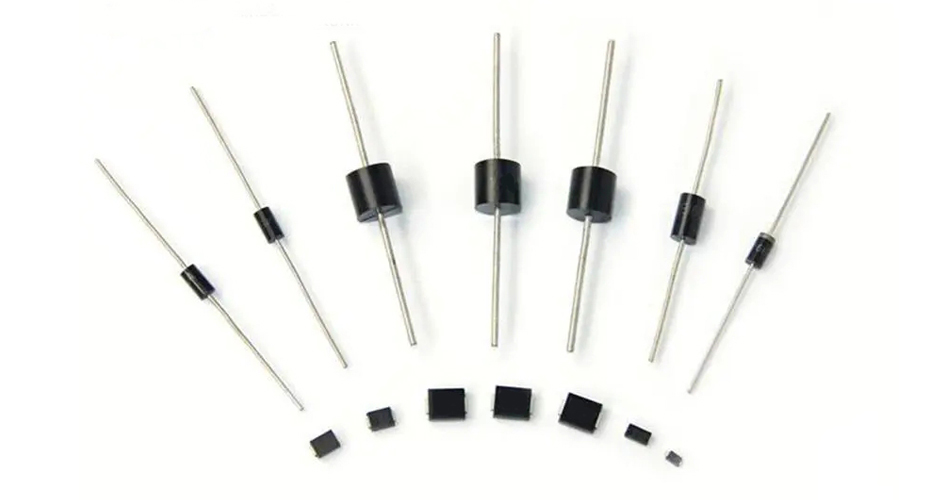1. Anti-revolution.

In the main circuit, a diode is connected in series, which makes use of the unidirectional conductivity of the diode to realize the simplest and reliable low-cost anti-reverse connection function circuit. This low-cost scheme is generally used in small current situations, such as small toys.

Because the diodes conduction will have a conduction voltage drop of 0.7V (silicon tube), if the actual current is large, it will produce a heat loss, which will lead to heating. Moreover, if the reverse connection voltage is large, exceeding the reverse cut-off voltage will also break down the diode itself, resulting in the diode failure, which can not play the function of anti-reverse connection, and thus can not play the role of protecting the subsequent circuit.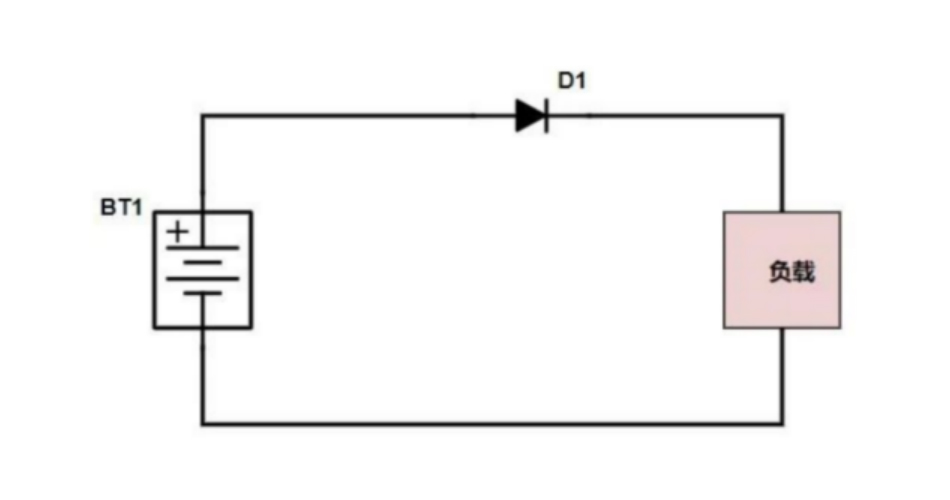2. Rectification

The function of the rectifier circuit is to convert the low voltage AC output from the AC step-down circuit into the unidirectional pulsating DC. This is the rectification process of the AC. The rectifier circuit is mainly composed of rectifier diodes. The voltage after the rectifier circuit is no longer AC voltage, but a mixed voltage containing DC voltage and AC voltage, which is conventionally called one-way pulsating DC voltage.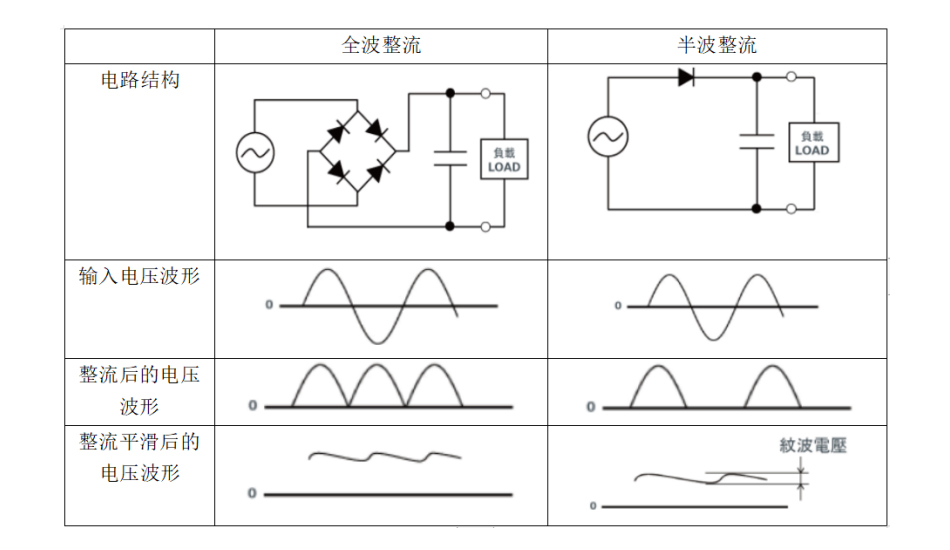3. Stabilized voltage

The diode with voltage stabilizing function is called zener diode, which is also called Zener diode in English. Using the reverse breakdown state of PN junction, its current can change in a large range while the voltage is basically unchanged.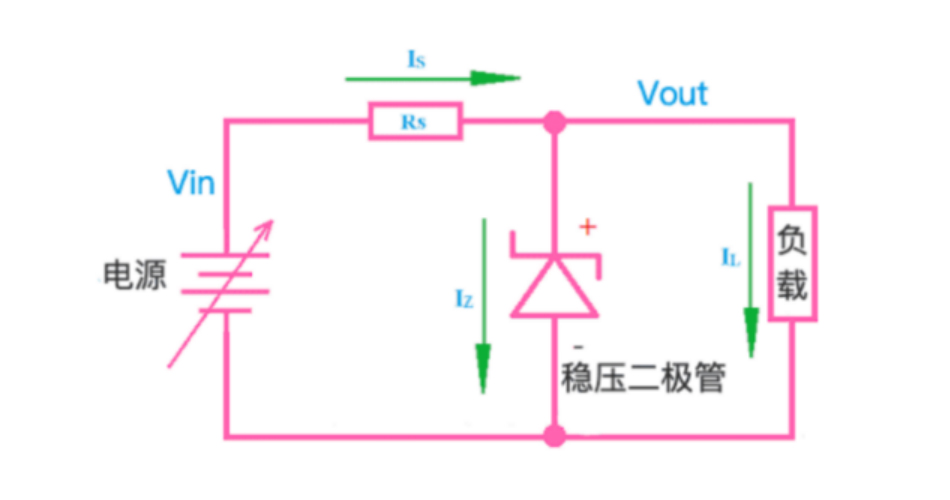4. Afterflow

The freewheeling diode is connected in parallel at both ends of the coil (inductive component). When the coil passes through the current, it will generate induced electromotive force at both ends. When the current disappears, its induced electromotive force will produce a reverse voltage on the elements in the circuit. When the reverse voltage is higher than the reverse breakdown voltage of the original, the original, such as the triode, will be damaged.

The freewheeling diodes are connected in parallel at both ends of the line. When the current flowing through the coil disappears, the induced electromotive force generated by the coil is consumed through the work of the circuit composed of the diode and the coil, which protects the safety of other components in the circuit. Or the freewheeling diode in the BUCK chip circuit.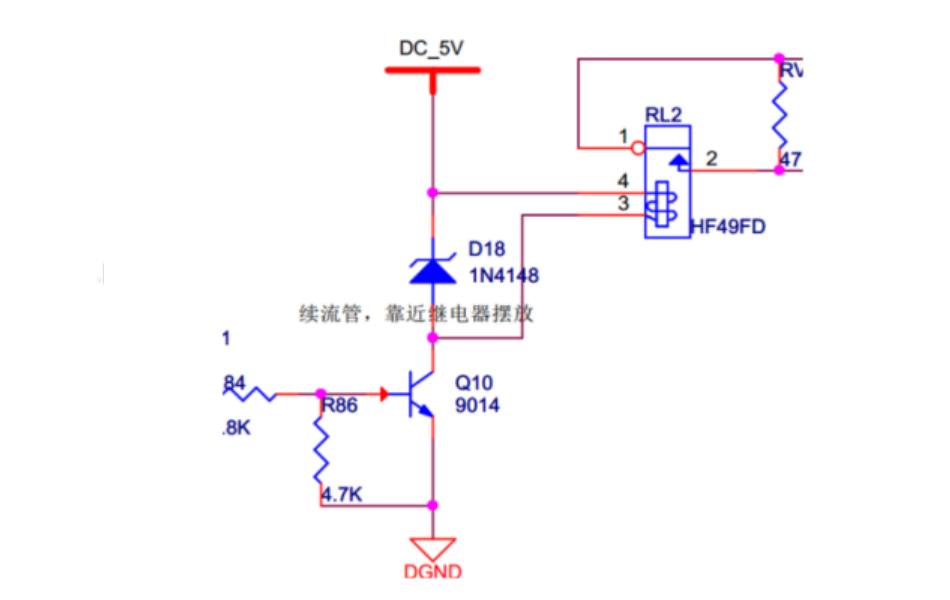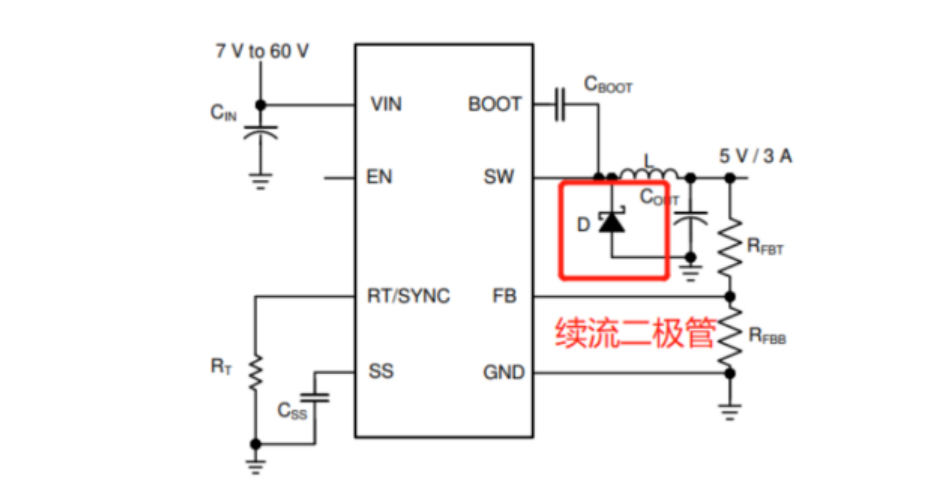5. Detection

The peak detection circuit detects the maximum value of the input signal amplitude. Its working principle is: when the input voltage amplitude is greater than the diode forward voltage, the diode is on, the output voltage is added to the capacitor C1, and both ends of the capacitor are charged. When the input voltage amplitude is lower than the previous input voltage amplitude, the diode is in the reverse bias cut-off state. At this time, the voltage at both ends of the capacitor is basically unchanged; If the signal is input again, the input voltage amplitude must be higher than the voltage at both ends of the capacitor at this time (that is, the forward voltage applied to the diodes) before the diode can be turned on.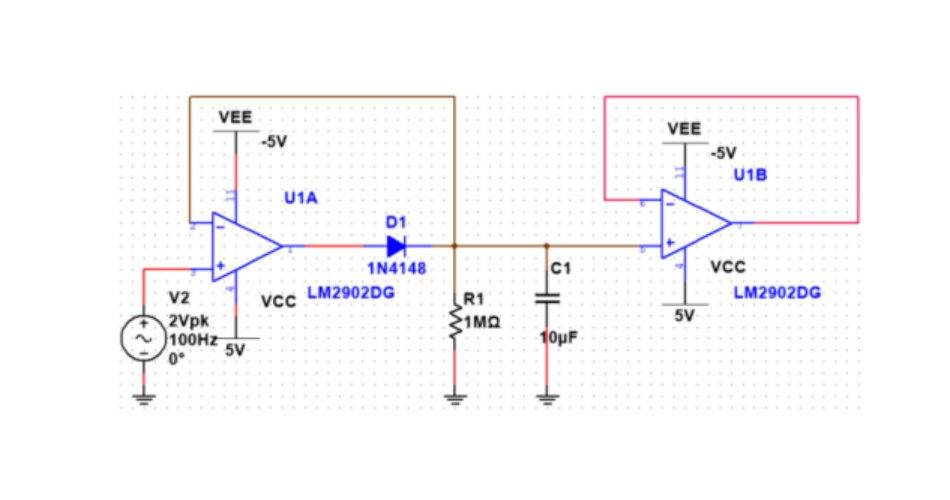6. Double pressure

The following figure is a schematic diagram of a double voltage circuit. Its working process is roughly analyzed as follows:

When the power supply is negative for half a cycle, diode D1 is on, D2 is off, the current flows from the lower end of the power supply through D1, and C1 returns to the power supply, so the capacitance C1 is positive on the right and negative on the left, as shown by the red arrow in the figure below. When the power supply is positive for half a cycle, the voltage on the capacitor C1 is superimposed with the power supply voltage to make the diode D2 turn on, the diode D1 turn off, the capacitor C2 is positive and negative, and the peak voltage can reach 2 times the peak voltage of the power supply, that is, double the voltage.

The current direction during this half cycle is shown by the orange arrow in the figure below: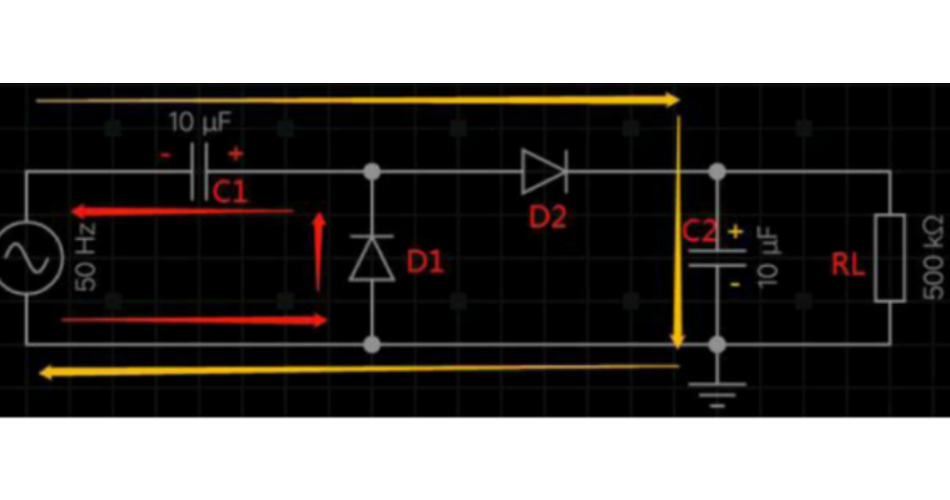7. Voltage clamping

In some ADC detection circuits, two diodes will be used for clamping protection. The principle is very simple. 0.7V is the conduction voltage drop of D1 and D2. When the voltage in Vin is greater than or equal to 3.3V+0.7V, D35 will be on and Vout will be clamped at 4V; When Vin is less than or equal to -0.7V, Vout is clamped at about -0.7V.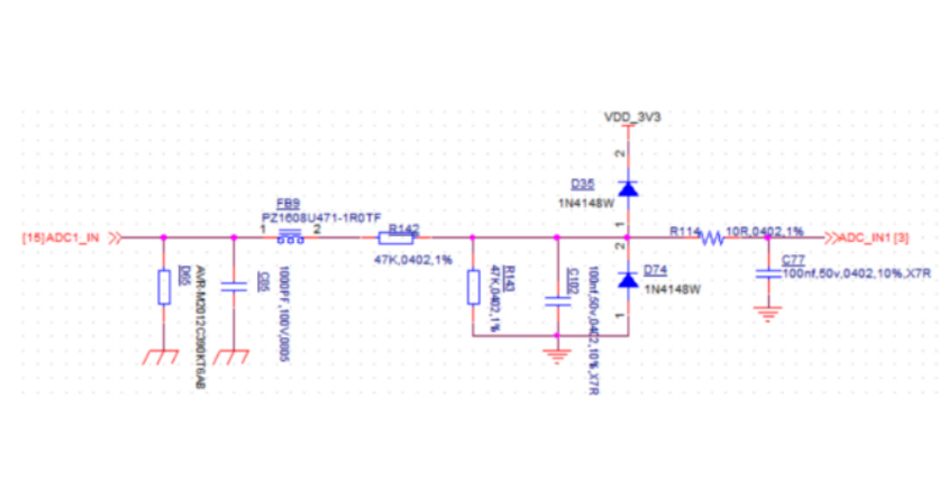8. Envelope detection

The circuit structure diodes is shown below. The key point of the design is that the time constant of RC diodes should be far greater than the period of carrier frequency and far less than the period of modulated signal.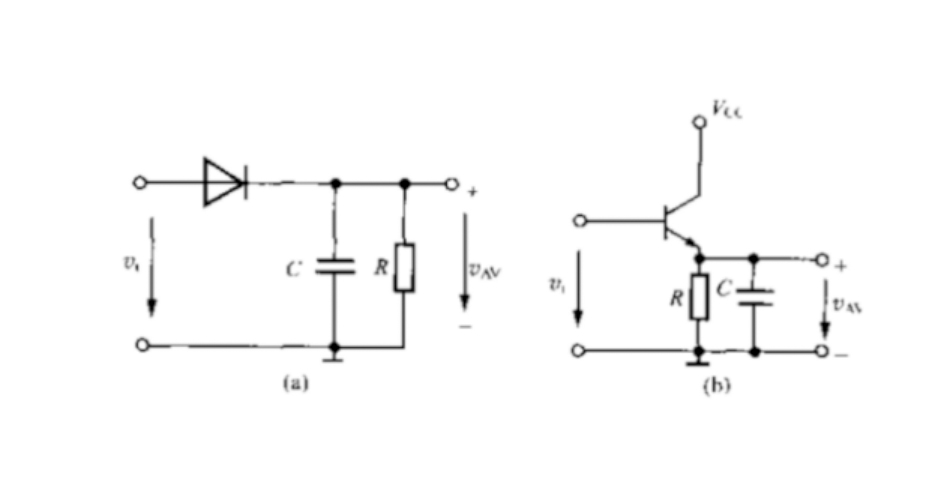### Which industries need pcba processing?### What is OEM One Stop?